## Past Courses of Prof. Dmitry Pelinovsky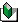Teaching Philosophy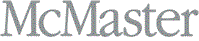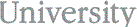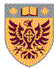### 2019-2020

 Methods of Applied Mathematics II (MATH 742)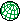Engineering Mathematics III (MATH 2Z03) This is mathematics course for second-year students in engineering programs. The course webpage features plan of lectures, class notes, and exams with solutions.Partial Differential Equations (MATH 3FF3) This is mathematics course for third-year students in applied mathematics. The course webpage features plan of lectures and home assignments with solutions.This is an applied mathematics course for graduate students. The course webpage features plan of lectures and home assignments with solutions.

### 2018-2019

 Methods of Applied Mathematics I (MATH 741)Engineering Mathematics III (MATH 2Z03) This is mathematics course for second-year students in engineering programs. The course webpage features plan of lectures, class notes, and exams with solutions.Partial Differential Equations (MATH 3FF3) This is mathematics course for third-year students in applied mathematics. The course webpage features plan of lectures and home assignments with solutions.This is an applied mathematics course for graduate students. The course webpage features plan of lectures and home assignments with solutions.

### 2017-2018

 Methods of Applied Mathematics I (MATH 741)PDEs for Engineering (MATH 3I03) This is mathematics course for third-year students in material engineering. The course webpage features plan of lectures, class notes, MATLAB programs, and home assignments with solutions.This is an applied mathematics course for graduate students. The course webpage features plan of lectures and home assignments with solutions.

### 2016-2017

 Advanced Differential Equations (MATH 3F03) Methods of Applied Mathematics II (MATH 742)Advanced Calculus I (MATH 2X03) This is mathematics course for second-year students from mathematics and physics programs. The course webpage features plan of lectures, MATLAB programs, and home assignments with solutions.This is an applied mathematics course for third- and final-year students. The course webpage features plan of lectures and home assignments with solutions.This is an applied mathematics course for graduate students. The course webpage features plan of lectures and home assignments with solutions.

### 2015-2016

 Mathematical Physics I (MATH 3C03) Advanced Differential Equations (MATH 3F03)This is an applied mathematics course for third-year physics students. The course webpage features plan of lectures and home assignments with solutions.This is an applied mathematics course for third- and final-year students. The course webpage features plan of lectures and home assignments with solutions.Complex Analysis II (MATH 4X03) This is the applied mathematics course for final-year undergraduate and first-year graduate students. The course webpage features plan of lectures, MATLAB programs, and home assignments with solutions.

### 2014-2015

 Mathematical Physics I (MATH 3C03) Numerical Explorations (MATH 3Q03)This is an applied mathematics course for third-year physics students. The course webpage features plan of lectures and home assignments with solutions.This is an applied mathematics course for third- and final-year students. The course webpage features plan of lectures and home assignments with solutions.Discrete Dynamical Systems and Chaos (MATH 3DC3) This is the applied mathematics course for third- and final-year students. The course webpage features plan of lectures, MATLAB programs, and home assignments with solutions.

### 2012-2013

 Calculus III (MATH 2A03) Numerical Explorations (MATH 3Q03)This is an applied mathematics course for second-year students from Physics. The course webpage features plan of lectures and home assignments with solutions.This is an applied mathematics course for third- and final-year students from Mathematics. The course webpage features plan of lectures and home assignments with solutions.Methods of Applied Mathematics II (MATH 742) This is the applied mathematics course for graduate students from Mathematics. The course webpage features plan of lectures and home assignments with solutions.

### 2011-2012

 Numerical Exploration (MATH 3Q03)This is an applied mathematics course for third- and final-year students from Mathematics. The course webpage features plan of lectures and home assignments with solutions.Methods of Applied Mathematics (MATH 742) This is the applied mathematics course for graduate students from Mathematics. The course webpage features plan of lectures and home assignments with solutions.

### 2010-2011

 Discrete Dynamical Systems and Chaos (MATH 3DC3)This is an applied mathematics course for third- and final-year students from Mathematics and Physics. The course webpage features plan of lectures and home assignments with solutions.Topics in Mathematical Physics (MATH 748) This is the applied mathematics course for graduate students from Mathematics. The course webpage features plan of lectures and home assignments with solutions.

### 2009-2010

 Partial Differential Equations (MATH 3FF3)This is an applied mathematics course for third- and final-year students from Mathematics and Physics. The course webpage features plan of lectures and home assignments with solutions.Advanced Differential Equations (MATH 743) This is the applied mathematics course for graduate students from Mathematics and Physics. The course webpage features plan of lectures and home assignments with solutions. The course is a beta-trial of the new textbook "Localization in periodic potentials".

### 2008-2009

 Linear Algebra II (MATH 2R03)This is the pure and applied mathematics course for second-year students from Mathematics and Physics. The course webpage features plan of lectures, problems for tutorials, and home assignments with solutions.Numerical Linear Algebra (MATH 2T03) This is the applied mathematics course for second-year students from Mathematics and Physics. The course webpage features plan of lectures, problems for tutorials, home assignments with solutions, and computer codes to the book "Numerical Mathematics".

### 2007-2008

 Methods of Applied Mathematics I (MATH 741) Numerical Methods for Differential Equations (MATH 4Q03)This is the applied mathematics course for graduate students from Mathematics. The course webpage features plan of lectures and assignments with solutions.This is the applied mathematics course for fourth-year and graduate students from Mathematics, Physics, and Engineering Physics. The course webpage features plan of lectures, problems for tutorials, home assignments with solutions, and computer codes to the book "Numerical Mathematics".Engineering Mathematics II (MATH 2M03) This is the engineering mathematics course for second-year students from Physics and Engineering. The course webpage features plan of lectures, problems for tutorials, and home assignments with solutions.

### 2005-2006

 Methods of Applied Mathematics I (MATH 741) Methods of Mathematical Physics II (MATH 3D03)This is the applied mathematics course for graduate students from Mathematics. The course webpage features plan of lectures and assignments with solutions.This is the applied mathematics course for third-year students from Mathematics, Physics, and Engineering Physics. The course webpage features plan of lectures, problems for tutorials, and home assignments with solutions.Linear Algebra I (MATH 1B03) This is the general mathematics course for first-year students from Mathematics, Science, and Engineering. The course webpage features online courseware, plan of lectures, problems for tutorials, and home assignments with solutions.

### 2004-2005

 Stability and Bifurcations (MATH 746) Methods of Mathematical Physics II (MATH 3D03)This is the applied mathematics course for graduate students from Mathematics. The course webpage features plan of lectures and assignments with solutions.This is the applied mathematics course for third-year students from Mathematics, Physics, and Engineering Physics. The course webpage features online courseware, plan of lectures, problems for tutorials, and home assignments with solutions.Methods of Mathematical Physics I (MATH 3C03) This is the applied mathematics course for third-year students from Mathematics, Physics, and Engineering Physics. The course webpage features online courseware, plan of lectures, problems for tutorials, home assignments with solutions, and links to interactive computer demos.

### 2003-2004

 Numerical Methods for Differential Equations (MATH 4Q03) Methods of Applied Mathematics II (MATH 742)This is the applied mathematics course for fourth-year students from Engineering Physics. The course webpage features plan of lectures, home assignments, solutions and results, and weblinks to interactive computer demos.This is the applied mathematics course for graduate students from Mathematics. The course webpage features plan of lectures, assignments and solutions, and weblinks to interactive computer demos.Methods of Mathematical Physics I (MATH 3C03) This is the applied mathematics course for third-year students from Mathematics, Physics, Engineering Physics and Medical Physics. The course webpage features plan of lectures, problems for tutorials, home assignments and solutions, links to interactive computer demos, and grade calculator.

### 2002-2003

 Partial Differential Equations I (MATH 3FF3) Numerical Methods for Differential Equations (MATH 4Q03)This is the general mathematics course for third-year students from Mathematics, Physics and Engineering. The course webpage features plan of lectures, home assignments and solutions, weblinks to interactive computer demos, and grade calculator.This is the applied mathematics course for fourth-year students from Engineering Physics. The course webpage features plan of lectures, home assignments and solutions, weblinks to interactive computer demos, and grade calculator.This is the applied mathematics course for fourth-year and graduate students from Mathematics. The course webpage features plan of lectures, assignments and solutions, weblinks to interactive computer demos, and grade calculator.Advanced Engineering Mathematics III (MATH 3J04) This is the applied mathematics course for third-year students from Civil Engineering. The course webpage features plan of lectures, problems for tutorials, assignments and solutions, and grade calculator.

### 2001-2002

 Partial Differential Equations I (MATH 3FF3) Scientific Computing (MATH 1J03)This is the general mathematics course for third-year students from Mathematics, Physics and Engineering. The course webpage features plan of lectures, assignments and solutions, quizzes and solutions, weblinks to interactive computer demos, and grade calculator.This is the applied mathematics course for first-year students from Mathematics, Physics and Computer science. The course webpage features interactive lecture notes in Word and HTML, interactive computer laboratories in HTML with codes in MATLAB, assignments and solutions, and grade calculator.Advanced Engineering Mathematics III (MATH 3J04) This is the applied mathematics course for third-year students from Civil Engineering. The course webpage features plan of lectures, problems for tutorials, assignments and solutions, grade calculator, and online student evaluations.

### 2000-2001Numerical Methods for Differential Equations (MATH 4Q03) This is the applied mathematics course for fourth-year students from Engineering Physics. The course webpage features interactive lecture notes, home assignments and solutions, grade calculator, on-line student evaluations, and WebCT login.Complex Analysis II (MATH 4X03) This is the pure mathematics course for fourth-year students from Mathematics. The course webpage features plan of lectures and home assignments.Advanced Engineering Mathematics III (MATH 3J04) This is the applied mathematics course for third-year students from Civil Engineering. The course webpage features plan of lectures, problems for tutorials, assignments and solutions, computer projects, grade calculator, and on-line student evaluations and comments.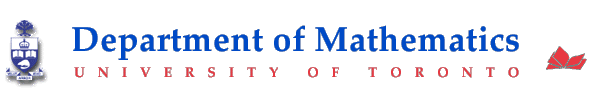### 1999-2000

 Calculus I for business and medicine (MAT 135Y)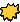Theory and Applications of Linear Programming and Network Flows (APM 261F) This is the applied mathematics course for second-year students from Computer Science. The course webpage features plan of lectures and on-line interactive quizzes.This is the basic mathematics course for first-year students from School of Business.

### 1998-1999

 Calculus I for business and medicine (MAT 135Y)Applications of Linear Programming (APM 235S) This is the applied mathematics course for summer students from Computer Science.This is the basic mathematics course for first-year students from School of Business.

### 1997-1998

 Applications of Linear Programming (APM 235W)Applications of Linear Programming (APM 235S) This is the applied mathematics course for summer students from Computer Science.This is the applied mathematics course for second-year students from Computer Science.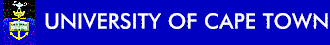### 1997

 Partial Differential Equations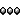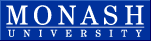### 1995-1996

 Calculus II